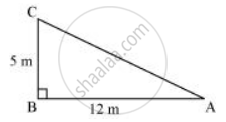Share

# A Tree is Broken at a Height of 5 M from the Ground and Its Top Touches the Ground at a Distance of 12 M from the Base of the Tree. Find the Original Height of the Tree. - CBSE Class 7 - Mathematics

ConceptRight-angled Triangles and Pythagoras Property

#### Question

A tree is broken at a height of 5 m from the ground and its top touches the ground at a distance of 12 m from the base of the tree. Find the original height of the tree.

#### SolutionIn the given figure, BC represents the unbroken part of the tree. Point C represents the point where the tree broke and CA represents the broken part of the tree. Triangle ABC, thus formed, is right-angled at B.

Applying Pythagoras theorem in ΔABC,

AC2 = BC2 + AB2

AC2 = (5 m)2 + (12 m)2

AC2 = 25 m2 + 144 m2 = 169 m2

AC = 13 m

Thus, original height of the tree = AC + CB = 13 m + 5 m = 18 m

Is there an error in this question or solution?

#### APPEARS IN

NCERT Solution for Mathematics for Class 7 (2018 to Current)
Chapter 6: The Triangle and its Properties
Ex. 6.50 | Q: 5 | Page no. 130
Solution A Tree is Broken at a Height of 5 M from the Ground and Its Top Touches the Ground at a Distance of 12 M from the Base of the Tree. Find the Original Height of the Tree. Concept: Right-angled Triangles and Pythagoras Property.
S